NCERT Solutions for Class 9 Maths Chapter 13 Surface Areas and Volumes Ex 13.2 are part of NCERT Solutions for Class 9 Maths. Here we have given NCERT Solutions for Class 9 Maths Chapter 13 Surface Areas and Volumes Ex 13.2.

 Board CBSE Textbook NCERT Class Class 9 Subject Maths Chapter Chapter 13 Chapter Name Surface Areas and Volumes Exercise Ex 13.2 Number of Questions Solved 11 Category NCERT Solutions

## NCERT Solutions for Class 9 Maths Chapter 13 Surface Areas and Volumes Ex 13.2

Question 1.
The curved surface area of a right circular cylinder of height 14 cm is 88 cm2. Find the diameter of the base of the cylinder.
Solution:
We have, height = 14 cm
Curved surface area Of a right circular cylinder = 88 cm2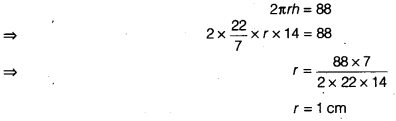r = 1 cm
Diameter = 2 x Radius = 2 x 1 = 2 cm

Question 2.
It is required to make a closed cylindrical tank of height 1 m and base diameter 140 cm from a metal sheet. How many square metres of the sheet are required for the same?
Solution:
Let r be the radius and h be the height of the cylinder.
Given, r = $$\frac { 140 }{ 2 }$$ = 70 cm = 0.70 m
and h = 1 m
Metal sheet required to make a closed cylindrical tank
= Total surface area
= 2πr (h + r)
= 2 x $$\frac { 22 }{ 7 }$$ x 0.7(1 + 0.70)
= 2 x 22 x 0.1 x 170 = 7.48m2
Hence, the sheet required to make a closed cylindrical tank = 7.48m2

Question 3.
A metal pipe is 77 cm long. The inner ft diameter of a cross section is 4 cm, the outer diameter being 4.4 cm (see figure). Find its
(i) inner curved surface area.
(ii) outer curved surface area.
(iii) total surface area.Solution:
We have, h = 77cm
Outer diameter (d1) = 4.4 cm
and inner diameter (d2) = 4 cm
Outer radius (r1) = 2.2 cm
(i) Inner curved surface area = 2πr2h = 2x $$\frac { 22 }{ 7 }$$ x 2 x 77 = 88 x 11 = 968 cm2
(ii) Outer curved surface area = 2πr1h
= 2 x $$\frac { 22 }{ 7 }$$ x 2.2 x 77
= 44 x 2.2 x 11= 1064.8cm2
(iii) Total surface area = Inner curved surface area + Outer curved surface area + Areas of two bases
= 968 + 1064.8 + 2$$\frac { 22 }{ 7 }$$ (r12 – r2)
= 968 + 1064.8 + 2 x $$\frac { 22 }{ 7 }$$ [(2.2) – r2]
= [2032.8 + 2 x $$\frac { 22 }{ 7 }$$ (4.84 – 4)]
= 2032.8 +$$\frac { 44 }{ 7 }$$ x 0.84 = 2032.8+ 44x 0.12
= 2032.8 + 5.28 cm2 = 2038.08 cm2

Question 4.
The diameter of a roller is 84 cm and its length is 120 cm. It takes 500 complete revolutions to move once over to level a playground. Find the area of the playground in m2.
Solution:
We have, diameter of a roller = 84 cm
r = radius of a roller = 42 cm
h = 120 cm
To cover 1 revolution = Curved surface area of roller
= 2πrh
= 2 x $$\frac { 22 }{ 7 }$$ x 42 x 120
= 44 x 720 cm2
= 31680 cm2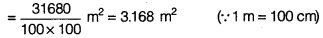Area of the playground = Takes 500 complete revolutions = 500 x 3.168 m2
= 1584 m2

Question 5.
A cylindrical pillar is 50 cm in diameter and 3.5 m in height. Find the cost of painting the curved surface of the pillar at the rate of ₹12.50 per m2.
Solution:
Given, Diameter = 50 cm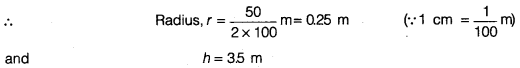Curved surface area of the pillar = 2πrh = 2 x $$\frac { 22 }{ 7 }$$ x 0.25 x 35
= 2 x 22 x 0.25 x 0.5 = 55 m2
Cost of painting per m2 = ₹ 12.50
Cost of painting 5.5 m2 = ₹ 12.50×5.5 = ₹ 68.75

Question 6.
Curved surface area of a right circular cylinder is 4.4 m2. If the radius of the base of the cylinder is 0.7 m, find its height.Curved surface area of a right circular cylinder is 4.4 m2. If the radius of the base of the cylinder is 0.7 m, find its height.
Solution:
We have, curved surface area of a right circular cylinder = 4.4m2
∴ 2πrh = 4.4Hence, the height of the right circular cylinder is 1 m

Question 7.
he inner diameter of a circular well is 3.5 m. It is 10 m deep. Find
(i) its inner curved surface area.
(ii) the cost of plastering this curved surface at the rate of ₹40 per m2.
Solution:
We have, inner diameter = 3.5 m
∴ inner radius = $$\frac { 3.5 }{ 2 }$$ m
and h= 10m
(i) Inner curved surface area = 2πrh = 2x $$\frac { 22 }{ 7 }$$ x $$\frac { 3.5 }{ 2 }$$ x10 = 22 x 5 = 110m2
(ii) Cost of plastering perm2 = ₹40
Cost of plastering 110 m2 = ₹40x 110= ₹4400

Question 8.
In a hot water heating system, there is a cylindrical pipe of length 28 m and diameter 5 cm. Find the total radiating surface in the system.
Solution:
We have, h = 28 m
Diameter = 5 cm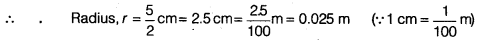Total radiating surface in the system = Curved surface area of the cylindrical pipe
= 2πrh =2 x $$\frac { 22 }{ 7 }$$ x 0.025 x 28 = 4.4 m2

Question 9.
Find
(i) the lateral or curved surface area of a closed cylindrical petrol storage tank that is 4.2 m in diameter and 4.5 m high.
(ii) how much steel was actually used, if $$\frac { 1 }{ 12 }$$ of the steel actually used was wasted in making the tank?
Solution:
(i) We have, diameter = 4.2 mSince, $$\frac { 1 }{ 2 }$$ of the actual steel used was wasted, therefore the area of the steel which was actually used for making the tankQuestion 10.
In figure, you see the frame of a lampshade. It is to be covered with a decorative cloth. The frame has a base diameter of 20 cm and height of 30 cm. A margin of 2.5 cm is to be given for folding it over the top and bottom of the frame. Find how much cloth is required for covering the lampshade.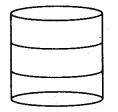Solution:
Given r = $$\frac { 20 }{ 2 }$$ cm = 10cm
h = 30 cm
Since, a margin of 2.5 cm is used for folding it over the top and bottom so the total height of frame,
h1 = 30 + 25 + 25
h1 = 35 cm
∴ Cloth required for covering the lampshade = Its curved surface area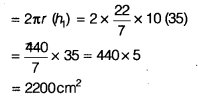Question 11.
The students of a Vidyalaya were asked to participate in a competition for making and decorating penholders in the shape of a cylinder with a base, using cardboard. Each penholder was to be of radius 3 cm and height 10.5 cm. The Vidyalaya was to supply the competitors with cardboard. If there were 35 competitors, how much cardboard was required to be bought for the competition?
Solution:
Cardboard required by each competitor
= Base area + Curved surface area of one penholder
= πr2 + 2πrh [Given, h = 10.5 cm, r = 13cm]
= $$\frac { 22 }{ 7 }$$ x (3)2 + 2 x $$\frac { 22 }{ 7 }$$ x 3 x 105
= (28.28 + 198) cm2
= 22828 cm2
For 35 competitors cardboard required = 35 x 22628 = 7920 cm2
Hence, 7920 cm2 of cardboard was required to be bought for the competition.

We hope the NCERT Solutions for Class 9 Maths Chapter 13 Surface Areas and Volumes Ex 13.2 help you. If you have any query regarding NCERT Solutions for Class 9 Maths Chapter 13 Surface Areas and Volumes Ex 13.2, drop a comment below and we will get back to you at the earliest.